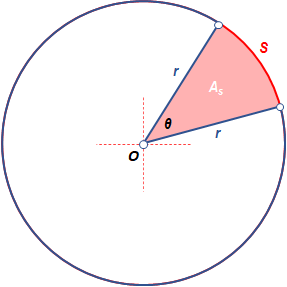# The area of a sector with a radius of 14 yards is 38.28 square yards. Calculate the approximate...

## Question:

The area of a sector with a radius of {eq}14 {/eq} yards is {eq}38.28 {/eq} square yards. Calculate the approximate angle of the sector. Round to the nearest tenth.

## Area of a Sector of a Circle

The figure below shows a sector that is defined as a pie-shaped segment of a circle. It is formed by enclosing of the two radii, {eq}r {/eq}, and an arc subtended by central angle, {eq}\theta {/eq}The area of this sector of a circle, {eq}A_{s} {/eq} can be determined by these formulas.

• If the central angle is measured in radians

• If the central angle is measured in degrees

Given the following dimensions of a sector of a circle.

We're required to determine for the measurement of the central angle, {eq}\theta {/eq}

From the formula for determining the area of a sector of a circle, we expressed the formula into {eq}\theta {/eq} in terms of {eq}r {/eq} and {eq}A{s} {/eq}

\begin{align} A_{s} &= \dfrac{\theta}{2} \times r^2 \\[0.3cm] \dfrac{\theta}{2} \times r^2 &= A_{s} \\[0.3cm] \theta r^2 &= 2 A_{s} \\[0.3cm] \dfrac{\theta r^2}{r^2} &= \dfrac{2 A_{s}}{r^2} \\[0.3cm] \theta &= \dfrac{2 A_{s}}{r^2} \\[0.3cm] \end{align} \\

Considering the simplified formula above, we substitute the given dimensions to solve for the measurement of central angle, {eq}\theta {/eq}

\begin{align} \theta &= \dfrac{2 (38.28\text{ yd}^2)}{(14\text{ yd})^2} \\[0.3cm] &= 0.3906122449\text{ rad} \\[0.3cm] \theta & \approx 0.4\text{ rad} \\[0.3cm] \end{align} \\

Therefore, the measure of the central angle is {eq}\bf{0.4\text{ rad}} {/eq}Sector of a Circle: Definition & Formula

from

Chapter 5 / Lesson 3
16K

In this lesson, we will discuss sectors of a circle and how to find the area of a sector. You will learn the different parts that make up a sector and test your skills with practice problems.